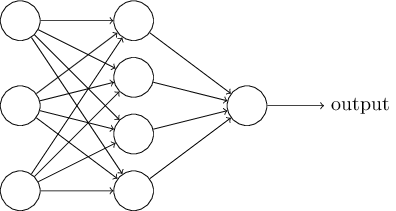# 1.4: The architecture of neural networks

$$\newcommand{\vecs}{\overset { \rightharpoonup} {\mathbf{#1}} }$$ $$\newcommand{\vecd}{\overset{-\!-\!\rightharpoonup}{\vphantom{a}\smash {#1}}}$$$$\newcommand{\id}{\mathrm{id}}$$ $$\newcommand{\Span}{\mathrm{span}}$$ $$\newcommand{\kernel}{\mathrm{null}\,}$$ $$\newcommand{\range}{\mathrm{range}\,}$$ $$\newcommand{\RealPart}{\mathrm{Re}}$$ $$\newcommand{\ImaginaryPart}{\mathrm{Im}}$$ $$\newcommand{\Argument}{\mathrm{Arg}}$$ $$\newcommand{\norm}{\| #1 \|}$$ $$\newcommand{\inner}{\langle #1, #2 \rangle}$$ $$\newcommand{\Span}{\mathrm{span}}$$ $$\newcommand{\id}{\mathrm{id}}$$ $$\newcommand{\Span}{\mathrm{span}}$$ $$\newcommand{\kernel}{\mathrm{null}\,}$$ $$\newcommand{\range}{\mathrm{range}\,}$$ $$\newcommand{\RealPart}{\mathrm{Re}}$$ $$\newcommand{\ImaginaryPart}{\mathrm{Im}}$$ $$\newcommand{\Argument}{\mathrm{Arg}}$$ $$\newcommand{\norm}{\| #1 \|}$$ $$\newcommand{\inner}{\langle #1, #2 \rangle}$$ $$\newcommand{\Span}{\mathrm{span}}$$$$\newcommand{\AA}{\unicode[.8,0]{x212B}}$$

In the next section I'll introduce a neural network that can do a pretty good job classifying handwritten digits. In preparation for that, it helps to explain some terminology that lets us name different parts of a network. Suppose we have the network:As mentioned earlier, the leftmost layer in this network is called the input layer, and the neurons within the layer are called input neurons. The rightmost or output layer contains the output neurons, or, as in this case, a single output neuron. The middle layer is called a hidden layer, since the neurons in this layer are neither inputs nor outputs. The term "hidden" perhaps sounds a little mysterious - the first time I heard the term I thought it must have some deep philosophical or mathematical significance - but it really means nothing more than "not an input or an output". The network above has just a single hidden layer, but some networks have multiple hidden layers. For example, the following four-layer network has two hidden layers:Somewhat confusingly, and for historical reasons, such multiple layer networks are sometimes called multilayer perceptrons or MLPs, despite being made up of sigmoid neurons, not perceptrons. I'm not going to use the MLP terminology in this book, since I think it's confusing, but wanted to warn you of its existence.

The design of the input and output layers in a network is often straightforward. For example, suppose we're trying to determine whether a handwritten image depicts a "9" or not. A natural way to design the network is to encode the intensities of the image pixels into the input neurons. If the image is a $$64$$ by $$64$$ greyscale image, then we'd have $$4,096 = 64×64$$ input neurons, with the intensities scaled appropriately between $$0$$ and $$1$$. The output layer will contain just a single neuron, with output values of less than $$0.5$$ indicating "input image is not a 9", and values greater than $$0.5$$ indicating "input image is a 9 ".

While the design of the input and output layers of a neural network is often straightforward, there can be quite an art to the design of the hidden layers. In particular, it's not possible to sum up the design process for the hidden layers with a few simple rules of thumb. Instead, neural networks researchers have developed many design heuristics for the hidden layers, which help people get the behaviour they want out of their nets. For example, such heuristics can be used to help determine how to trade off the number of hidden layers against the time required to train the network. We'll meet several such design heuristics later in this book.

Up to now, we've been discussing neural networks where the output from one layer is used as input to the next layer. Such networks are called feed forward neural networks. This means there are no loops in the network - information is always fed forward, never fed back. If we did have loops, we'd end up with situations where the input to the σσ function depended on the output. That'd be hard to make sense of, and so we don't allow such loops.

However, there are other models of artificial neural networks in which feedback loops are possible. These models are called recurrent neural networks. The idea in these models is to have neurons which fire for some limited duration of time, before becoming quiescent. That firing can stimulate other neurons, which may fire a little while later, also for a limited duration. That causes still more neurons to fire, and so over time we get a cascade of neurons firing. Loops don't cause problems in such a model, since a neuron's output only affects its input at some later time, not instantaneously.

Recurrent neural nets have been less influential than feedforward networks, in part because the learning algorithms for recurrent nets are (at least to date) less powerful. But recurrent networks are still extremely interesting. They're much closer in spirit to how our brains work than feedforward networks. And it's possible that recurrent networks can solve important problems which can only be solved with great difficulty by feedforward networks. However, to limit our scope, in this book we're going to concentrate on the more widely-used feedforward networks.

This page titled 1.4: The architecture of neural networks is shared under a CC BY-NC 3.0 license and was authored, remixed, and/or curated by Michael Nielson via source content that was edited to the style and standards of the LibreTexts platform; a detailed edit history is available upon request.